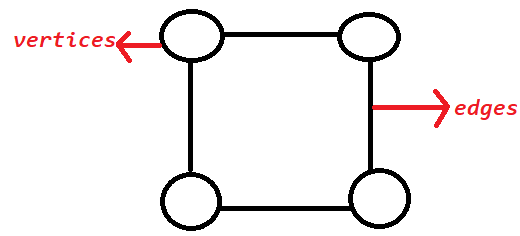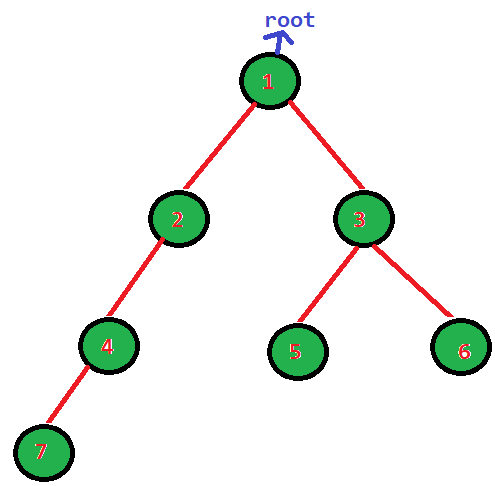# Graph And Tree

## Introduction

What do you understand by graphs and trees? You might have listened to these terms other than in coding, but it plays an essential role in the technical rounds of FAANG companies. So these two topics are a must-do for the interviews.

graph is a non-linear data structure used to represent the networks, and it consists of vertices(V), or it can be called nodes and edges(E) that connect the pair of nodes.

• In the graph, the edge can be directed or bidirected.
• It can be weighted.There are two commonly used representations of a graph are:

The other vital non-linear data structure is trees that offer relationships between the nodes in a structure and consist of nodes and edges, which arranges data items in sorted order and cannot be in the form of a cycle and should always be connected unlike graphs.

There are several types of trees, such as a binary tree, binary search tree, AVL tree, threaded binary tree, B-tree, etc.

• The topmost node is the tree's root, and all other nodes connected to it are its children(sub-nodes).
• It does not contain any loop.## FAQ’S

1). What is the key difference between graph and tree?

A graph can have loops or self-loops, whereas trees cannot have any loops.

A graph can have connected or disconnected components, whereas nodes in the tree should be connected.

2). What are the methods to traverse a graph?

There are two methods to traverse a graph: Depth First Search(DFS) and Breadth-First Search(BFS).

3). Difference between DFS and BFS?

DFS uses Stack data structure, and BFS uses Queue data structure.

The Time complexity of BFS is O(V + E) when Adjacency List is used and O(V^2) when Adjacency Matrix is used.

The Time complexity of DFS is also O(V + E) when Adjacency List is used and O(V^2) when Adjacency Matrix is used.

## Key Takeaway

In this blog, we have covered the two non-linear data structures and how they differ in their properties.

Graphs and trees are used to solve various complex problems in competitive programming. The graph is a data structure where vertices are connected using edges and form a cycle. Whereas Trees is a data structure where the topmost node is the tree's root, and all others are its children, it cannot have a cycle.

Don't stop here; prepare the topic trees and graph in-depth for product-based companies' technical rounds.

You can use CodeStudio to practice various DSA questions typically asked in interviews of your placements. It will help you in acquring efficient coding techniques.